• argmax
千次阅读
2022-02-27 08:31:57
def argmax(a, axis=None, out=None, *, keepdims=np._NoValue):
"""
Returns the indices of the maximum values along an axis.

Parameters
----------
a : array_like
Input array.
axis : int, optional
By default, the index is into the flattened array, otherwise
along the specified axis.
out : array, optional
If provided, the result will be inserted into this array. It should
be of the appropriate shape and dtype.
keepdims : bool, optional
If this is set to True, the axes which are reduced are left
in the result as dimensions with size one. With this option,
the result will broadcast correctly against the array.

Returns
-------
index_array : ndarray of ints
Array of indices into the array. It has the same shape as a.shape
with the dimension along axis removed. If keepdims is set to True,
then the size of axis will be 1 with the resulting array having same
shape as a.shape.

--------
ndarray.argmax, argmin
amax : The maximum value along a given axis.
unravel_index : Convert a flat index into an index tuple.
take_along_axis : Apply np.expand_dims(index_array, axis)
from argmax to an array as if by calling max.

Notes
-----
In case of multiple occurrences of the maximum values, the indices
corresponding to the first occurrence are returned.

Examples
--------
>>> a = np.arange(6).reshape(2,3) + 10
>>> a
array([[10, 11, 12],
[13, 14, 15]])
>>> np.argmax(a)
5
>>> np.argmax(a, axis=0)
array([1, 1, 1])
>>> np.argmax(a, axis=1)
array([2, 2])

Indexes of the maximal elements of a N-dimensional array:

>>> ind = np.unravel_index(np.argmax(a, axis=None), a.shape)
>>> ind
(1, 2)
>>> a[ind]
15

>>> b = np.arange(6)
>>> b = 5
>>> b
array([0, 5, 2, 3, 4, 5])
>>> np.argmax(b)  # Only the first occurrence is returned.
1

>>> x = np.array([[4,2,3], [1,0,3]])
>>> index_array = np.argmax(x, axis=-1)
>>> # Same as np.amax(x, axis=-1, keepdims=True)
>>> np.take_along_axis(x, np.expand_dims(index_array, axis=-1), axis=-1)
array([,
])
>>> # Same as np.amax(x, axis=-1)
>>> np.take_along_axis(x, np.expand_dims(index_array, axis=-1), axis=-1).squeeze(axis=-1)
array([4, 3])

Setting keepdims to True,

>>> x = np.arange(24).reshape((2, 3, 4))
>>> res = np.argmax(x, axis=1, keepdims=True)
>>> res.shape
(2, 1, 4)
"""
kwds = {'keepdims': keepdims} if keepdims is not np._NoValue else {}
return _wrapfunc(a, 'argmax', axis=axis, out=out, **kwds)


返回沿轴的最大值的索引。

参数

----------

a:array_like

输入数组。

轴：int，可选

默认情况下，索引被放入展平数组中，否则

沿着指定的轴。

输出：阵列，可选

如果提供，结果将插入到此数组中。它应该

具有适当的形状和类型。

keepdims:bool，可选

如果设置为True，则缩小的轴将保留

结果为尺寸为1的尺寸。有了这个选择,，

结果将针对阵列正确广播。

例子：

>>> a = np.arange(6).reshape(2,3) + 10
>>> a
array([[10, 11, 12],
[13, 14, 15]])
>>> np.argmax(a)
5
>>> np.argmax(a, axis=0)
array([1, 1, 1])
>>> np.argmax(a, axis=1)
array([2, 2])

Indexes of the maximal elements of a N-dimensional array:

>>> ind = np.unravel_index(np.argmax(a, axis=None), a.shape)
>>> ind
(1, 2)
>>> a[ind]
15

>>> b = np.arange(6)
>>> b = 5
>>> b
array([0, 5, 2, 3, 4, 5])
>>> np.argmax(b)  # Only the first occurrence is returned.
1

>>> x = np.array([[4,2,3], [1,0,3]])
>>> index_array = np.argmax(x, axis=-1)
>>> # Same as np.amax(x, axis=-1, keepdims=True)
>>> np.take_along_axis(x, np.expand_dims(index_array, axis=-1), axis=-1)
array([,
])
>>> # Same as np.amax(x, axis=-1)
>>> np.take_along_axis(x, np.expand_dims(index_array, axis=-1), axis=-1).squeeze(axis=-1)
array([4, 3])

Setting keepdims to True,

>>> x = np.arange(24).reshape((2, 3, 4))
>>> res = np.argmax(x, axis=1, keepdims=True)
>>> res.shape
(2, 1, 4)python 机器学习 人工智能
更多相关内容
• numpy.argmax(array, axis) 用于返回一个numpy数组中最大值的索引值。当一组中同时出现几个最大值时，返回第一个最大值的索引值。 在运算时，相当于剥掉一层中括号，返回一个数组，分为一维和多维。一维数组剥掉一层...
• 从最简单的例子出发 假定现在有一个数组a = [3, 1, 2, 4, 6, 1]现在要算数组a中最大数的索引是多少.这个问题对于刚学编程的同学就能解决....但是可以帮助我们理解argmax. 解释 还是从一维数组出发.看下面的例子. impo
• ARGMAX是结构化模型的开源实现； 条件随机场和结构支持向量机。开源软件
• 这组函数有效地计算向量和矩阵的最大值/最小值参数。 它的核心是一个通过编译C源代码获得的MEX函数。 在演示中展示了它对 Matlab 内置 max 和 min 函数的好处。matlab
• function I = argmax(X, DIM)
• 0.0952, 0.1285, 0.5760, 0.1223], dtype=torch.float64) torch.Size() torch.DoubleTensor ''' argmax 寻找参数最大值的索引，但是该过程不可导 soft-argmax 为了得到一个向量中最大值所处的位置，我们利用此...

## softmax

Softmax函数是一个非线性转换函数，通常用在网络输出的最后一层，输出的是概率分布（比如在多分类问题中，Softmax输出的是每个类别对应的概率），计算方式如下：得到的是第i个位置对应的概率,每个位置的概率之和为1（可以看出Softmax仅进行计算，没有需要学习的参数）。

## Pytorch softmax API

torch.nn.functional.softmax(input, dim=None, _stacklevel=3, dtype=None)

input是我们输入的数据，dim是在哪个维度进行Softmax操作（如果没有指定，默认dim=1）

## softmax code example

• numpy 实现
import numpy as np
data = np.array([0.1, 0.3, 0.6, 2.1 ,0.55])
a = np.exp(data)/np.sum(np.exp(data))
print(a)
print(sum(a))
'''输出
[0.07795756 0.09521758 0.12853029 0.57603278 0.12226179]
0.9999999999999999
'''


向量中的参数被归一化，总和为1

• pytorch 实现
import torch
import torch.nn.functional as F
t_data = torch.from_numpy(data)
print(t_data)
print(t_data.shape)
print(t_data.type())

prob = F.softmax(t_data,dim=0) # dim = 0,在列上进行Softmax;dim=1,在行上进行Softmax
print(prob)
print(prob.shape)
print(prob.type())

'''output
tensor([0.1000, 0.3000, 0.6000, 2.1000, 0.5500], dtype=torch.float64)
torch.Size()
torch.DoubleTensor
tensor([0.0780, 0.0952, 0.1285, 0.5760, 0.1223], dtype=torch.float64)
torch.Size()
torch.DoubleTensor
'''


## argmax

寻找参数最大值的索引，但是该过程不可导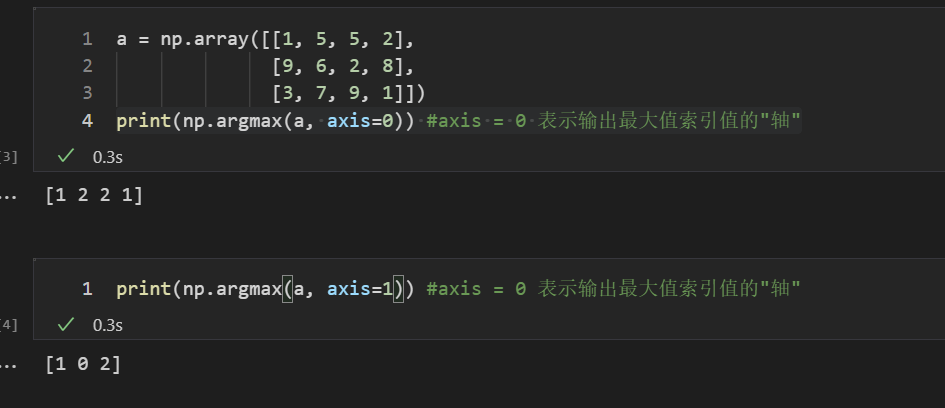## soft-argmax

为了得到一个向量中最大值所处的位置，我们利用此函数。但是这个函数不可导，所以无法计算其梯度。然而我们可以利用软化的max函数来计算，就是softmax。利用softmax，我们可以得到每个元素正则化后的值。此向量（）分布）的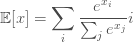np.sum(np.exp(data)/np.sum(np.exp(data)) * np.array([0,1,2,3,4]))    # E = p*index
'''output:
2.5694236670240085
'''


而最大之所在的位置应该是3。
从上面看到位置计算不够准确，一个原因就是最大值的概率不够大，或者说增大相对最大值而减弱其他值的影响就可以得到更加准确的位置坐标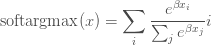可以看到，上式与softmax的期望只有一个差别，即给向量的每个元素乘以beta。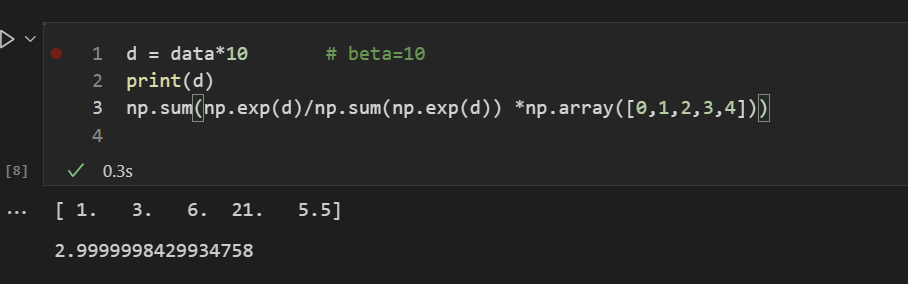输出的坐标为2.99，即为3，且这种寻找极值所在位置（坐标）的方法是可微的。常用于图像特征点位置的提取。

此外，LIFT原文3.5节提到：softargmax作为非极大值抑制NMS的可微分版本。就是说可以利用softargmax来替代NMS。

## 参考文档

展开全文pytorch 深度学习 softmax
• softmax，argmax，soft-argmax 理解_道长的博客-CSDN博客 1）softmax： 定义：就相当于密度函数。 特点：面积加起来等于1。 成效：向量中的参数被归一化，总和为1。 公司： 代码： import numpy ...

# 1）softmax：

定义：就相当于密度函数。

特点：面积加起来等于1。

成效：向量中的参数被归一化，总和为1。

公司：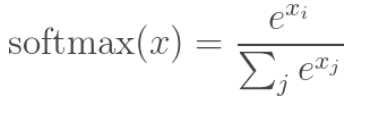代码：

import numpy as np
data=
展开全文pytorch
• torch.argmax()，如下图所示： 将输入input张量，无论有几维，首先将其reshape排列成一个一维向量，然后找出这个一维向量里面最大值的索引。 4. torch.argmax(input,dim) 函数解析 函数返回其他所有维在这个维度...

# 一. torch.argmax()函数解析

## 1. 官网链接

torch.argmax()，如下图所示：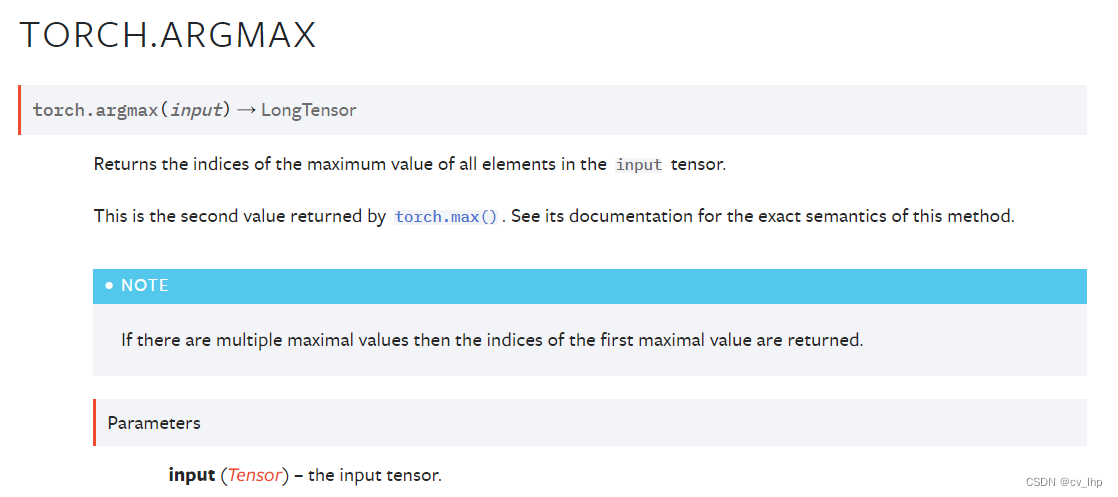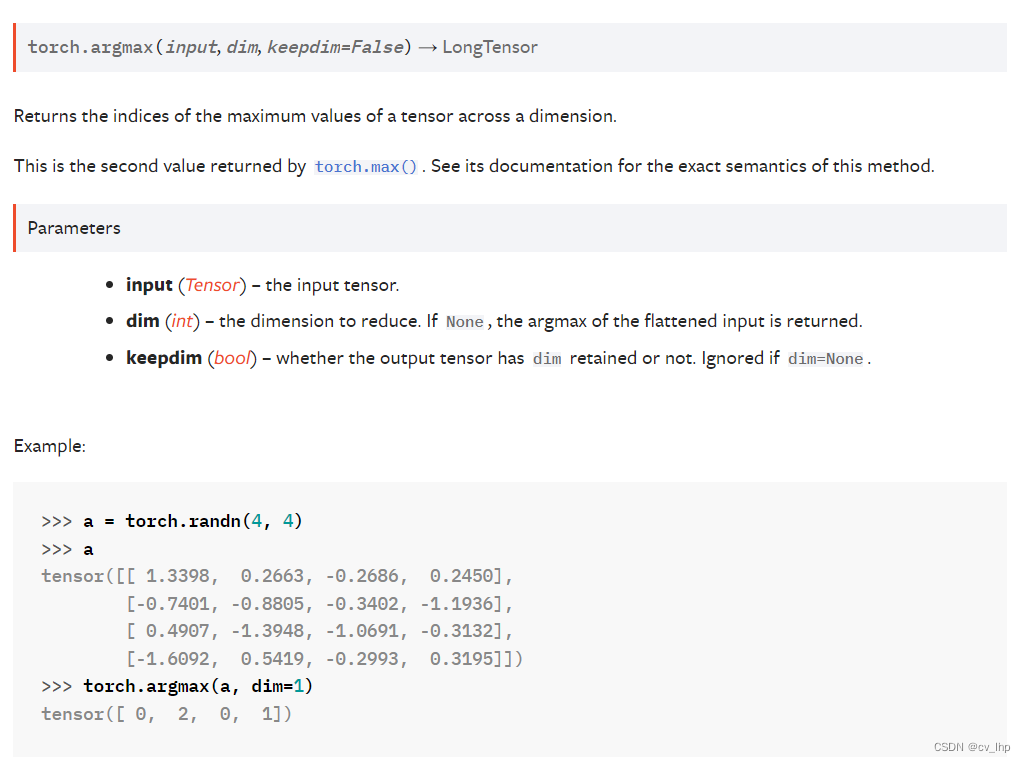## 2. torch.argmax(input)函数解析

torch.argmax(input) → LongTensor


将输入input张量，无论有几维，首先将其reshape排列成一个一维向量，然后找出这个一维向量里面最大值的索引。

## 3. 代码举例

import torch
x = torch.randn(3,4)
y = torch.argmax(x)#对应于x中最大元素的索引值
x,y

输出结果如下：
import torch
x = torch.randn(3,4)
y = torch.argmax(x)#对应于x中最大元素的索引值
x,y


## 4. torch.argmax(input,dim) 函数解析

torch.argmax(input, dim, keepdim=False) → LongTensor


函数返回其他所有维在这个维度上面张量最大值的索引。
torch.argmax()函数中dim表示该维度会消失，可以理解为最终结果该维度大小是1，表示将该维度压缩成维度大小为1。

举例理解：对于一个维度为(d0,d1) 的矩阵来说，dim=1表示求每一行中最大数的在该行中的列号，最后得到的就是一个维度为(d0,1) 的二维矩阵，最终列这一维度大小为1就要消失了，最终结果变成一维张量(d0)；
dim=0表示求每一列中最大数的在该列中的行号，最后我们得到的就是一个维度为(1，d1) 的二维矩阵，结果行这一维度大小为1就要消失了，最终结果变成一维张量(d1)。

因此，我们想要求每一行最大的列标号，我们就要指定dim=1，表示我们不要列了，保留行的size就可以了。
假如我们想求每一列的最大行标，就可以指定dim=0，表示我们不要行了，求出每一列的最大值的下标，最后得到（1,d1）的一维矩阵。

## 5. 代码举例

5.1 输入二维张量torch.Size([3, 4])，dim=0表示将dim=0这个维度大小由3压缩成1，然后找到dim=0这三个值中最大值的索引，这个索引表示dim=0行索引标号，结果张量维度变为torch.Size()。

import torch
x = torch.randn(3,4)
y = torch.argmax(x,dim=0)#dim=0表示将dim=0这个维度大小由3压缩成1，然后找到dim=0这三个值中最大值的索引，这个索引表示dim=0行索引标号
x,x.shape,y,y.shape

输出结果如下：
(tensor([[ 2.6347,  0.6456, -1.0461, -1.5154],
[-1.3955, -1.2618, -0.5886, -0.5947],
[-1.5272, -2.0960,  0.9428, -0.9532]]),
torch.Size([3, 4]),
tensor([0, 0, 2, 1]),
torch.Size())


5.2 输入二维张量torch.Size([3, 4])，dim=1表示将dim=1这个维度大小由4压缩成1，然后找到dim=1这四个值中最大值的索引，这个索引表示dim=1列索引标号，结果张量维度变为torch.Size()。

import torch
x = torch.randn(3,4)
y = torch.argmax(x,dim=1)#dim=1表示将dim=1这个维度大小由4压缩成1，然后找到dim=1这四个值中最大值的索引，这个索引表示dim=1列索引标号
x,x.shape,y,y.shape

输出结果如下：
(tensor([[ 0.1549,  0.4331,  0.3575,  1.1077],
[ 2.0233,  2.0085, -0.6101, -1.8547],
[-0.5101, -0.4052,  0.3458, -0.7802]]),
torch.Size([3, 4]),
tensor([3, 0, 2]),
torch.Size())


5.3 输入三维张量torch.Size([2, 3, 4])，dim=0表示将dim=0这个维度大小由2压缩成1，然后找到dim=0这两个值中最大值的索引，这个索引表示dim=0维索引标号。dim=0,即将第一个维度消除，也就是将两个矩阵只保留一个，因此要在两组中作比较，即将上下两个的矩阵分别在对应的位置上比较大小，结果矩阵张量维度变为torch.Size([3, 4])。

import torch
x = torch.randn(2,3,4)
y = torch.argmax(x,dim=0)#dim=0表示将dim=0这个维度大小由2压缩成1，然后找到dim=0这两个值中最大值的索引，这个索引表示dim=0维索引标号
x,x.shape,y,y.shape

输出结果如下：
(tensor([[[-1.4430,  0.0306, -1.0396,  0.1219],
[ 0.1016,  0.0889,  0.8005,  0.3320],
[-1.0518, -1.4526, -0.4586, -0.1474]],

[[ 1.2274,  1.5806,  0.5444, -0.3088],
[-0.8672,  0.3843,  1.2377,  2.1596],
[ 0.0671,  0.0847,  0.5607, -0.7492]]]),
torch.Size([2, 3, 4]),
tensor([[1, 1, 1, 0],
[0, 1, 1, 1],
[1, 1, 1, 0]]),
torch.Size([3, 4]))


5.4 输入三维张量torch.Size([2, 3, 4])，dim=1表示将dim=1这个维度大小由3压缩成1，然后找到dim=1这三个值中最大值的索引，这个索引表示dim=1维索引标号。dim=1，即将第二个维度消除（纵向压缩成一维），结果矩阵张量维度变为torch.Size([2, 4])。

import torch
x = torch.randn(2,3,4)
y = torch.argmax(x,dim=1)#dim=1表示将dim=1这个维度大小由3压缩成1，然后找到dim=1这三个值中最大值的索引，这个索引表示dim=1维索引标号
x,x.shape,y,y.shape

输出结果如下：
(tensor([[[-1.7136,  0.5528,  0.5171,  1.2978],
[ 1.0250, -0.2687,  0.6727, -0.2013],
[ 0.1366, -1.0563,  0.1965,  1.5303]],

[[-0.0048,  1.6265, -1.0341, -0.3994],
[ 1.5536,  0.9739, -0.0913,  0.0889],
[-0.6703, -0.9099, -0.6400, -0.1807]]]),
torch.Size([2, 3, 4]),
tensor([[1, 0, 1, 2],
[1, 0, 1, 1]]),
torch.Size([2, 4]))


5.5 输入三维张量torch.Size([2, 3, 4])，dim=2表示将dim=2这个维度大小由4压缩成1，然后找到dim=2这四个值中最大值的索引，这个索引表示dim=2维索引标号。dim=2,即将第三个维度消除（横向压缩成一维），结果矩阵张量维度变为torch.Size([2, 3])。

import torch
x = torch.randn(2,3,4)
y = torch.argmax(x,dim=2)#dim=2表示将dim=2这个维度大小由4压缩成1，然后找到dim=2这四个值中最大值的索引，这个索引表示dim=2维索引标号
x,x.shape,y,y.shape

输出结果如下：
(tensor([[[-0.3493,  0.8838,  0.5876, -0.3967],
[-1.5795,  2.6964,  0.7266,  0.3517],
[-0.6949, -1.4385, -0.0993,  0.1679]],

[[-0.4924, -0.8955,  0.5511,  0.6287],
[ 0.2338, -0.5787, -0.2081, -1.3032],
[ 0.6429,  0.0949,  0.3319, -0.8551]]]),
torch.Size([2, 3, 4]),
tensor([[1, 1, 3],
[3, 0, 0]]),
torch.Size([2, 3]))

展开全文pytorch 深度学习 python 神经网络
• 在写AI算法的Demo时，偶然间出现了一个bug，发现是我不小心将tf.argmax()写成了np.argmax()，正好闲来无事，辨析下两个API的使用 一、np.argmax()的使用 np.argmax()是Python的第三方库numpy中的一个常见API，经常...python tensorflow 深度学习 numpy
• 文章目录导读官方文档地址参数解析举例...今天的主角是torch.argmax(input, dim, keepdim=False)。 官方文档地址 https://pytorch.org/docs/stable/generated/torch.argmax.html torch.argmax(input) → LongTensorpytorch 深度学习
•python 开发语言
• ## torch.argmax()函数

千次阅读 2022-03-08 16:57:06
argmax函数：torch.argmax(input, dim=None, keepdim=False) 返回指定维度最大值的序号，dim给定的定义是：the demention to reduce，就是把dim这个维度，变成这个维度的最大值的index。 1）dim表示不同维度。特别的...pytorch 深度学习 机器学习 神经网络
• ## argmax()函数

万次阅读 多人点赞 2022-02-08 11:47:09
根据定义，argmax()是一种函数，是对函数求参数(集合)的函数，也就是求自变量最大的函数。 或者根据名字来理解，arg是argument的缩写，argument是作为函数输入的值，也就是自变量，argmax就是求自变量最大值。 当...机器学习 深度学习 自然语言处理
•pytorch
• tf.argmax(data, axis=None) 用tensorflow 做 mnist分类时，用到这个接口，于是就研究了下这个接口的用法： 如果是一维数组呢？ data = tf.constant([1,2,3]) with tf.Session() as sess: print(sess.run(tf.argmax...tensorflow 深度学习 机器学习
• torch.Tensor.argmax(dim:int,keepdim:bool)->Tensor 找Tensor中的指定维度dim上最大值所在下标，返回是一个Tensor。keepdim指定返回的Tensor是否和原来的一样形状。若为False，则在dim维度上被压缩。 (只返回...pytorch python 机器学习
• numpy中的argmax简而言之就是返回最大值的索引，当使用np.argmax(axis)，这里方向axis的指定往往让人不理解。 简而言之：这里axis可以让我们从行、列、或者是深度方向来看一个高维数组。 二、代码实验 1、一维数组python 机器学习
• 目录一、前言二、函数讲解1、argmax（）函数2、参数1）a2）axis（可选）3）out（可选）3、返回值4、注意 一、前言 在数组里查找相同元素，返回索引的时候用到了该函数 二、函数讲解 1、argmax（）函数 老样子，我们...学习 python 算法
• 今天需要给大家介绍两个函数，argMin(),argMax() argMax():计算 ‘arg’ 最大值 ‘val’ 价值。 如果有几个不同的值 ‘arg’ 对于最大值 ‘val’，遇到的第一个值是输出。argMin() 与argMax() 的功能正好是相反的，...clickhouse
• $\mathop{\arg\max}\limits_{\theta}$latex
• argmax()返回的就是最大数的索引 argmax()有一个参数axis，可以指定函数返回不同维的最大值。 一维 import numpy as np a = np.array([1, 2, 3, 4, 5]) print(np.argmax(a, axis=0)) 结果是4。而在python中一维...python 机器学习
• argmax返回的只是indexes。 import torch import numpy as np if __name__ == '__main__': a = torch.tensor([[1,2,3], [4,5,6]]) b = a.max(dim=1) c = a.max(dim=1) d = a.argmax(dim=1) b = b.repytorch 深度学习
• torch.max(x , 1)返回两个结果， 第一个是最大值，第二个是对应的索引值； 第二个参数 0 代表按列取最大值并返回对应的行索引值，1 代表按行取最大值并返回对应的列索引值。 torch.max(x , 1)返回一个结果，返回最大...pytorch 深度学习 机器学习
• torch.argmax 函数详解1. 函数介绍2. 实例演示 1. 函数介绍 torch.argmax(input, dim=None, keepdim=False) 返回指定维度最大值的序号 dim给定的定义是：the demention to reduce.也就是把dim这个维度的，变成这个...pytorch
• 1、numpy之argmax()作用 返回相应维度axis上的最大值的位置。 2、具体应用 深度学习图像分割多分类最后是softmax得分结果，我们需要将这个浮点型的结果保存成整型的标签图像，这样才算完成图像分割。softmax结果转...
• softmax 输入为向量，输出为值为0-1之间的向量，和为1。在分类任务中作为概率出现在交叉熵损失函数中。 import numpy as np data=np.array([0.1, 0.3, 0.6, 2.1 ,0.55]) np.exp(data)/np.sum(np.exp...argmax 为了机器学习 python 深度学习
• y=argmax f(t) ：y是f(t)函数取到最大值时的参数t 比如有 f（t）=t^2 ； t的取值范围{0,1,2}，那么： y=max f(t)=4； y=argmax f(t)=2； ———————————————— 版权声明：本文为CSDN博主「马里奥...
• ## argmax()、max()介绍

千次阅读 2021-03-28 17:09:38
argmax()、max()的介绍 在开始之前先明确“行轴”和“列轴”的方向如上图。 对张量的操作 import torch t = torch.tensor([[3,4,1,0],[12,1,2,3],[52,34,88,11]]) t # 输出为： tensor([[ 3, 4, 1, 0], [12, 1, 2,......